# 7.NS.A.1b / 7.NS.A.1c - Addition and Subtraction of Rational Numbers (Part 1 of 2)

##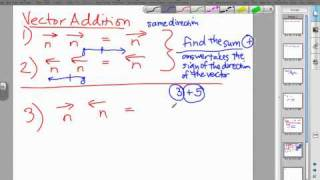By MathwithMrAlmeida

Watch Mr. Almeida fully explain how to add and subtract rational numbers using vectors and the concept of a number line.# Absolute Value and Additive Inverse | 7.NS.A.1 | 7th Grade Math

##By ParksMath# Division of Rational Numbers

##By Khan Academy

This video walks through the division of 4-5 rational numbers and includes a word problem.# Comparing rational numbers

##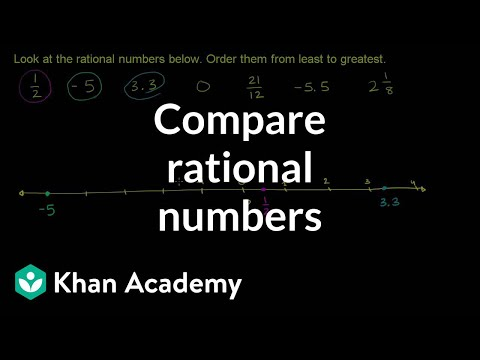By Khan Academy

U09_L1_T3_we4 Comparing Rational Numbers# Passive Sentences (Part 1)

##By Smrt English

Mark demonstrates how to make a sentence in the passive voice using 'be + past participle'.# The Active & Passive Voices #1

##By Smrt English

This lesson explains the reasons and ways to use the active and passive voices. Passive sentences shift the focus from the subject to the object. Passive sentences are used when the agent (doer of the action) is not important or unknown or the agent is clear.# Passive Sentences (Part 2)

##By Smrt English

Mark explains the difference between the active voice and the passive voice. He provides reasons why people use the passive voice.# The Active & Passive Voices #2

##By Smrt English

This lesson provides more detailed information about the active and passive voices. Passive sentences are used when it is unnecessary to say "by" an agent and is used as a past participle adjective. Active sentences can be used when speaking in casual situations when the subject is "you" or "we".# Dividing mixed numbers

##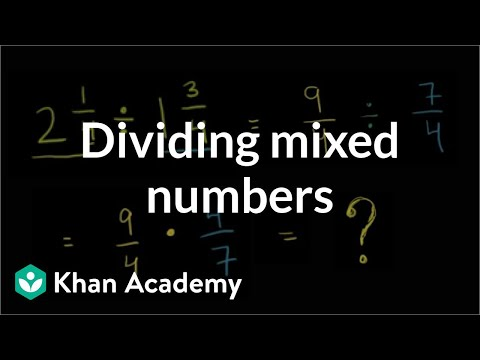By Khan Academy

Dividing Mixed Numbers# Multiplying and dividing negative numbers

##By Khan Academy

Find the product of numbers with different signs.# Multiplying and dividing negative numbers

##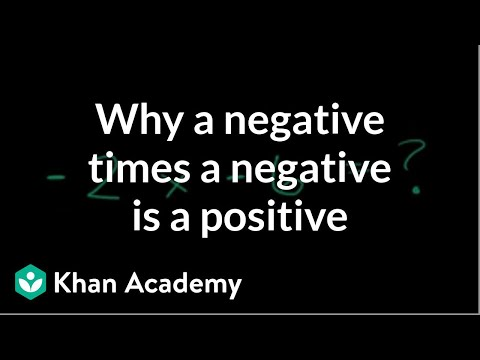By Khan Academy

Use the distributive property to understand the products of negative numbers.# Multiplying and dividing negative numbers

##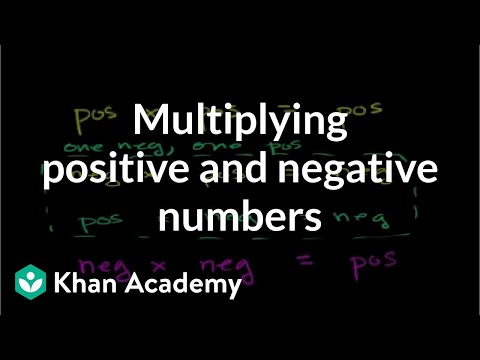By Khan Academy

Learn some rules of thumb for multiplying positive and negative numbers.# Multiplying and dividing negative numbers

##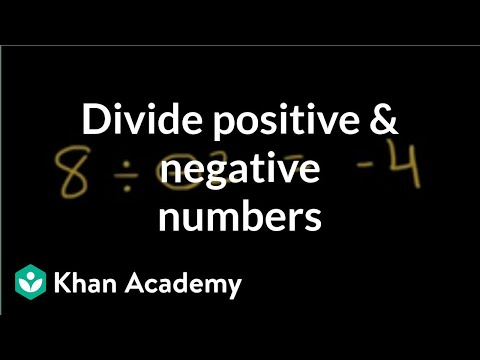By Khan Academy

Discover the basics of dividing with negative numbers.ï¿½ï¿½ï¿½ï¿½ï¿½ï¿½ï¿½ï¿½ï¿½ï¿½ï¿½ï¿½# Multiplying and dividing negative numbers

##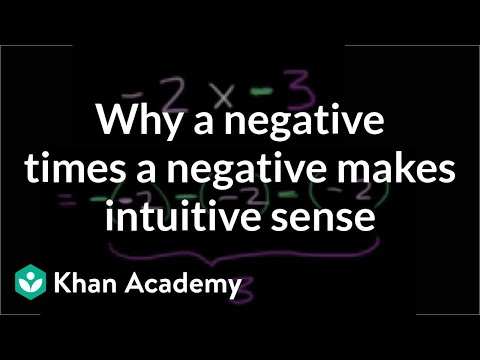By Khan Academy

Use the repeated addition model of multiplication to give an understanding of multiplying negative numbers.# Classifying numbers

##By Khan Academy

Learn how to classify numbers as whole numbers, integers, rational numbers, and irrational numbers.# Subtracting negative numbers intro

##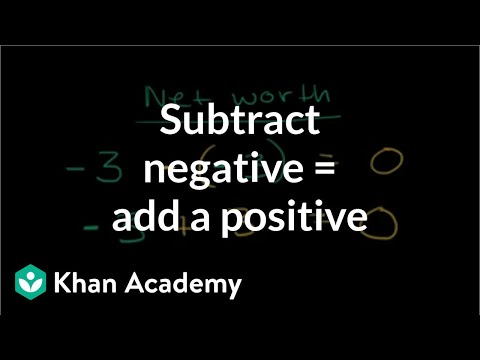By Khan Academy

Find out why subtracting a negative number is the same as adding the absolute value of that number.# Subtracting negative numbers intro

##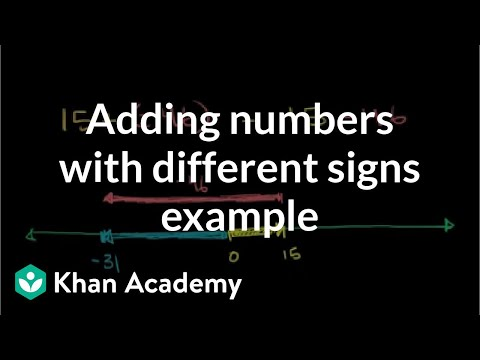By Khan Academy

Use a number line to add 15 + (-46) + 29# Adding negative numbers intro

##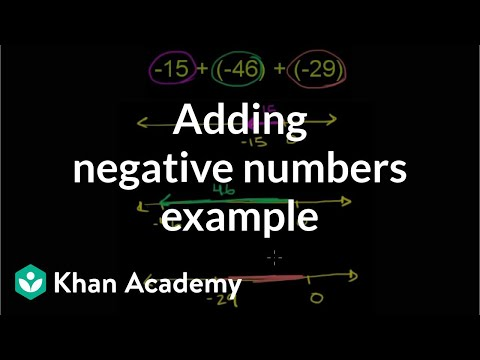By Khan Academy

Use a number line to add -15 + (-46) + (-29)# Subtracting negative numbers intro

##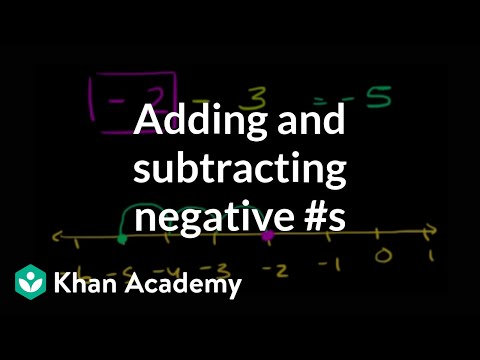By Khan Academy

Learn how to add and subtract negative numbers. The problems solved in this video are 2 - 3 = -1 and -2 - 3 = -5 and -2 + 3 = 1 and 2 - (-3) = 5 and -2 - (-3).# CA Algebra I: Number Properties and Absolute Value

##By Khan Academy

1-7 number properties and absolute value equations. All Khan Academy content is available for free at www.khanacademy.org